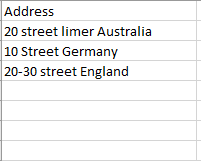# String manuplation in data table

Hi,

I am extracting data using data scrapping method from a website and storing in var Dt. For each row in dt i need to compare the street No with a VarA. Eg: VarA contains number 20, the row in Dt always starts with the street No Eg: 20 street limer Australia 3207. How do i write the code. Also the row in dt can also be eg: 10-30 street limer Australia 3207. In such cases how do i write the code.

I need to check if the street No matches.

Hey @RACHEL_PAUL ,
What if your Var value is 10 and the row has 10-30 ?
Should it consider the row or ignore?

Also please share sample input file if possible

Regards,

Hi @RACHEL_PAUL ,

Does 20 street limer Australia 3207 and 10-30 street limer Australia 3207 denote the same address?

Regards,

Hey @RACHEL_PAUL ,
If that is the case then you can try this

Note : I have assumed that the input will be like belowRegards,

Hi jithesh,

There is a possibility that the data be as 10-30 in such scenarios will this code run?

Hi Vishal,

Yes that’s right. It could also range as 10-100 or 10-200.

@RACHEL_PAUL , it would be helpful for us to find a solution if you can explain how 20 can also be represented as 10-30 in the address. Is there some specific criteria?

Regards,

@RACHEL_PAUL ,
Yes if your VarA is 10 then According to the code it will retrieve the first 2 digits from the currentrow by doing `Left(CurrentRow("Address").ToString,2)` which will return value as 10
then using if condition both the values will match .

Regards,

Hi vishal,

I am supoosed to find the addresses. So there is a possibility that the street no and the VarA will be the same or if street No will be in range that is (eg: 10-30) so if Var A that is (eg:20) is found between the range i need to consider that address.

1 Like

@RACHEL_PAUL ,
You mean if varA is 20 and the range is 10-30 then also it shopuld consider?

Regards,

Yes jitesh thats right.

Check the following expression, it will give you the datatable after filtering if the varA value is in the address:

``````dt.AsEnumerable.Where(function(x) if(x("Address").ToString.split(" "c).First.ToString.Split("-"c).Count>1,Enumerable.Range(Cint(x("Address").ToString.split(" "c).First.ToString.Split("-"c)(0).ToString),Cint(x("Address").ToString.split(" "c).First.ToString.Split("-"c)(1))).Contains(varA),x("Address").ToString.Substring(0,varA.ToString.Length).Equals(varA.ToString))).CopyToDataTable
``````

Regards,

@RACHEL_PAUL ,
Then please ignore the previous answer and continue with the @vishal.kp 's Solution.

Regards,

1 Like

Should this be in If condition?

@RACHEL_PAUL ,
No use a Assign Activity ,
(Left Side) Your Output Data Table = (Right side) The Above Code

The filtered data will be stored in the your output DataTable.

Regards,

1 Like

If you require that address to be extracted then:

``````dt.AsEnumerable.Where(function(x) if(x("Address").ToString.split(" "c).First.ToString.Split("-"c).Count>1,Enumerable.Range(Cint(x("Address").ToString.split(" "c).First.ToString.Split("-"c)(0).ToString),Cint(x("Address").ToString.split(" "c).First.ToString.Split("-"c)(1))).Contains(varA),x("Address").ToString.Substring(0,varA.ToString.Length).Equals(varA.ToString))).CopyToDataTable.Rows(0)("Address").ToString
``````

Before using the above expression, use a if condition and check the count is greater than 0, else it will throw error if there are no rows to copy to datatable:

``````dt.AsEnumerable.Where(function(x) if(x("Address").ToString.split(" "c).First.ToString.Split("-"c).Count>1,Enumerable.Range(Cint(x("Address").ToString.split(" "c).First.ToString.Split("-"c)(0).ToString),Cint(x("Address").ToString.split(" "c).First.ToString.Split("-"c)(1))).Contains(varA),x("Address").ToString.Substring(0,varA.ToString.Length).Equals(varA.ToString))).Count>0
``````

Regards,

This worked.

Thank you vishal and jitesh.

2 Likes

This topic was automatically closed 3 days after the last reply. New replies are no longer allowed.